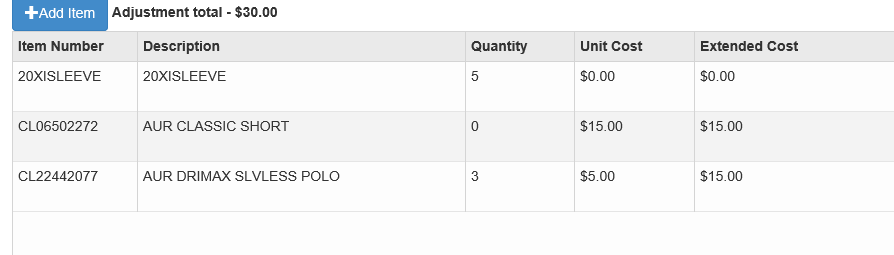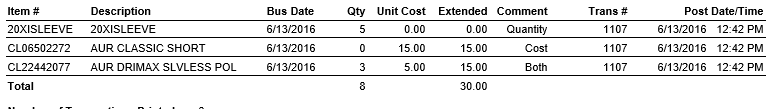# How do I make "Inventory" adjustments

There are 3 types of inventory adjustments (sample picture follows):

• Quantity Only
• Cost Only
• Both Quantity and CostQuantity Only

This would be used when the quantity in the system is wrong but the extended price is correct.  For instance, a cost of 20XISleeve should be 33.00 each and we have 10 on hand.  Our inventory cost shows \$330.00 but our quantity shows 5, this would require a quantity increase of 5. Click the Add Item button,  select the item, enter 5 (enter a negative sign in front to decrease the quantity) in the Item Quantity Box and leave the Item Cost at \$0.00.  See row 1 in the picture above.

Cost Only

This would be used when the extended cost in the system is wrong but the quantity on hand is correct.  For instance, a cost of AUIR Classic Shorts should be should \$18.00 each and we have 10 on hand.  In this case the extended cost should be \$180.00 but it is only \$165.00. This would require a cost increase of \$15.00. Click the Add Item button,  select the item, leave the Item Quantity at 0 and enter \$15.00 (enter a negative sign in front to decrease the cost) in the Item Cost. See row 2 in the picture above.

Both Quantity and Cost

This rare instance is where you do not want to use "Receipts" but you need to adjust both the quantity and the cost.  In our example above, Auir DriMax quantity is low by a count of 3 and the cost is off \$15.00.  Click the Add Item button,  select the item, the Item Quantity would be 3 and enter \$5.00 in the Item Cost.  The extended cost will be \$15.00 (3 times \$5.00). See row 3 in the picture above.

If you need to decrease the quantity AND the cost, be sure to put the negative in the quantity only.  The negative quantity times a positive item cost will equal a negative extended cost adjustment.• Review the onscreen adjustment, especially the extended cost.  Be sure the extended cost equals what you anticipate the amount should be.  Edit the adjustment until it matches what you expect
• Immediately review the Inventory Valuation and determine if the quantity and extended cost are correct.  If it is not correct, go back and make an adjustment.
• Consider adjusting cost or quantity only, it is much easier.